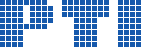Polish Information Processing Society# Parthood and Convexity as the Basic Notions of a Theory of Space

## Klaus Robering

Citation: Proceedings of the LQMR 2015 Workshop, Tomasz Lechowski, Przemysław Wałęga, Michał Zawidzki (eds). ACSIS, Vol. 7, pages 4154 ()

Full text

Abstract. A deductive system of geometry is presented which is based on atomistic mereology (''mereology with points'') and the notion of convexity. The system is formulated in a liberal many-sorted logic which makes use of class-theoretic notions without however adopting any comprehension axioms. The geometry developed within this framework roughly corresponds to the``line spaces'' known from the literature. The basic ideas of the system are presented in the article's``Introduction'' within a historical context. After a brief presentation of the logical and mereological framework adopted, a ``pregeometry'' is described in which only the notion of convexity but no further axiom is added to that background framework. Pregeometry is extended to the full system in three steps. First the notion of a line segment is explained as the convex hull of the mereological sum of two points. In a second step two axioms are added which describe what it means for a thus determined line segment to be ``straight''. In the final step we deal with the order of points on a line segment and define the notion of a line. The presentation of the geometric system is concluded with a brief consideration of the geometrical principles known by the names of Peano and Pasch. Two additional topics are treated in short sections at the end of the article: (1) the introduction of coordinates and (2) the idea of a ``geometrical algebra''.